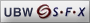ISSN 2167-5163
MathJax is on Publications results for "Anywhere=(ultraintuit*)"

### Citations

From Reviews: 0

MR0432418 (55 #5406) Reviewed
Geiser, James R.
A formalization of Essenin-Volpin's proof theoretical studies by means of nonstandard analysis.
J. Symbolic Logic 39 (1974), 81–87.
02E05 (02D99 02H25)Publication Year 1974 Review Published1978-03-01

Since the appearance of A. S. Esenin-Volʹpin's original papers on ultraintuitionism [Infinitistic methods (Proc. Sympos. Foundations of Math., Warsaw, 1959), pp. 201–223, Pergamon, Oxford, 1961; MR0147389 (26 #4905); Intuitionism and proof theory (Proc. Conf., Buffalo, N.Y., 1968), pp. 3–45, North-Holland, Amsterdam, 1970; MR0295876 (45 #4938)] there have been attempts to capture some of his ideas by more conventional mathematical means. Examples of such attempts include A. Ehrenfeucht's paper [Proceedings of the Tarski Symposium (Proc. Sympos. Pure Math., Vol. XXV, Univ. California, Berkeley, Calif., 1971), pp. 265–268, Amer. Math. Soc., Providence, R.I., 1974; MR0357078 (50 #9546)] and the reviewer's work [J. Symbolic Logic 36 (1971), 494–508; MR0304152 (46 #3287)]. For a nontechnical discussion of some of the philosophical issues, see M. Dummett's paper [Synthese 30 (1975), 301–324].
The present paper belongs to this trend, but is more directly pertinent to Esenin-Volʹpin's own motivations in that it tackles the question of a consistency proof for ZF, the principal intended application of ultraintuitionism. (The paper considers not ZF itself but an equiconsistent nonextensional theory ZF^{\text{}$^{\text{}$ and a variant (ZF)$(\text{ZF}^-)^\sim$.)
The first two pages give us a nice introduction to a portion of Esenin-Volʹpin's own philosophy, explaining how it differs from traditional intuitionism and describing the crucial (for this paper) notion of natural number sequences of different lengths. As pointed out by G. Kreisel in his review of Esenin-Volʹpin's first paper [Zbl 134, 9] above, this notion is reminiscent of nonstandard analysis; there also one deals with two systems N$N$, N${}^\ast N$ of natural numbers, of which only one, however, is standard.
Taking this analogy seriously, the author constructs two structures Tn0,T0${}^\ast\scr T_{n_0},\scr T_0$ the first of which satisfies all the axioms of ZF^{\text{}$^{\text{}$ without the axiom of infinity, whereas the second does satisfy the axiom of infinity but fails replacement and comprehension. A collection G$\scr G$ of proof trees is then set up in order to pick and choose between the properties of the two structures described above, so as to obtain the whole of (ZF)$(\text{ZF}^-)^\sim$ without obtaining, also, a contradiction.
The methods are ingenious and the treatment elegant, but unfortunately the author chooses to follow Esenin-Volʹpin's own, rather sketchy, style of exposition. Details of proofs are never given and even definitions and axioms are quite frequently omitted. There is no discussion, as there ought to be, of how the proposed consistency proof meets the conditions imposed by Gödel's second theorem. Thus the reader is intrigued and involved without quite knowing just what it is that he has learned. A fuller version of the paper is promised on p. 87, but has not been forthcoming. Surely, fifteen years after the initial announcements and claims, the mathematical community deserves something a bit more solid.
Reviewed by R. Parikh